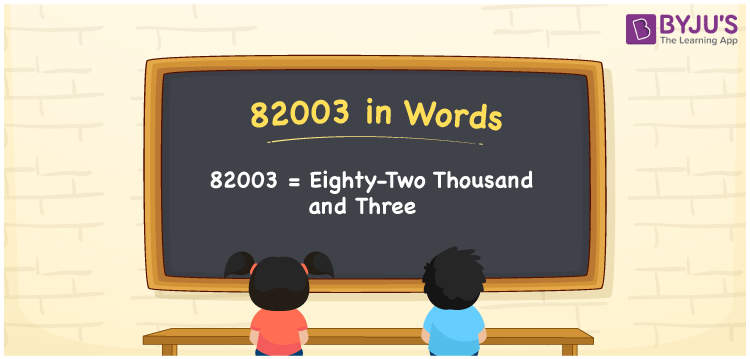# 82003 in Words

82003 in words is written as Eighty-two thousand three. In both the International System of Numerals and the Indian System of Numerals, 82003 is written as Eighty-two thousand three. The number 82003 is a Cardinal Number as it denotes some quantity. For example, “the boat costs 82003 rupees”.

 82003 in Words Eighty-two thousand three Eighty-two thousand three in Number 82003

## 82003 in English Words

82003 in English words is read as “Eighty-two thousand three”.## How to Write 82003 in Words?

To write 82003 in words, we shall use the place value chart. In the place value chart, put 8 in the ten thousands, 2 in the thousands, 0 in the hundreds and tens, and 3 in the ones. Let us make a place value chart to write the number 82003 in words.

 Ten Thousands Thousands Hundreds Tens Ones 8 2 0 0 3

Thus, we can write the expanded form as

8 × Ten Thousand + 2 × Thousand + 0 × Hundred + 0 × Ten + 3 × One

= 8 × 10000 + 2 × 1000 + 0 × 100 + 0 × 10 + 3 × 1

= 80000 + 2000 + 0 + 0 + 3

= 82003

= Eighty-two thousand three.

82003 is a natural number, the successor of 82002 and the predecessor of 82004.

82003 in words – Eighty-two thousand three

• Is 82003 an odd number? – Yes
• Is 82003 an even number? – No
• Is 82003 a perfect square number? – No
• Is 82003 a perfect cube number? – No
• Is 82003 a prime number? – Yes
• Is 82003 a composite number? – No

## Frequently Asked Questions on 82003 in Words

Q1

### How to write 82003 in words?

82003 in words is written as Eighty-two thousand three.
Q2

### How to write 82003 in the International and Indian System of Numerals?

In both, the system of numerals, 82003 in words, is written as Eighty-two thousand three.
Q3

### How to write 82003 in a place value chart?

In the place value chart, write 8 in the ten thousands, 2 in the thousands, 0 in the hundreds and tens and 3 in the ones, respectively.